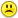+0

# (4+5)3 = 4(3)+ 5(3)

0
420
3

(4+5)3 = 4(3)+ 5(3)

Apr 10, 2015

#3
+5

Hey Saseflower, you made a boo booThey are equal

Apr 11, 2015

#1
0

What's the question?!

Apr 10, 2015
#2
+5

(4 + 5)3 = 4(3) + 5(3)

Use the distributive property of multiplication, and multiply

12 + 14 = 12 + 15

26 = 27

Those don't equal each other, so I usually put a slash through the =

Apr 10, 2015
#3
+5
Hey Saseflower, you made a boo boo